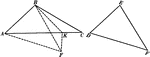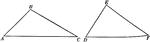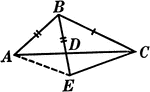### 2 Triangles Theorem

Illustration used to prove that "If two triangles have two sides of one equal respectively to two sides…### 2 Triangles Theorem

Illustration used to prove that "If two triangles have two sides of one equal respectively to two sides…### Segments Labeled In A Triangle

Illustration of triangle ABC with BE extended through the triangle at point D. Segment AB is equal to…